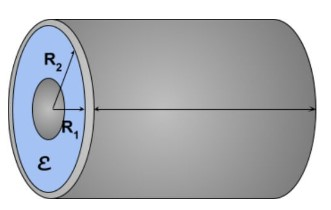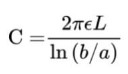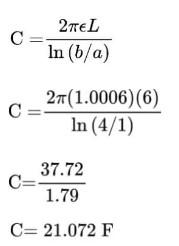# Cylindrical Capacitor Calculator

Input permittivity, length, outer & inner diameter of the conductor, and press calculate button to find capacitance  using cylindrical capacitor calculator

Formula:
C = 2 π ε LIn (b/a)
F/m
m
m
m

Give Us Feedback

## How to use the cylindrical capacitor calculator?

Follow these steps to use this tool to calculate the capacitance of a cylindrical capacitor.

1. Choose the quantity to calculate I.e capacitance.
2. Enter the permittivity.
3. Input the value of the length of the conductor.
4. Enter the values of inner and outer cylinders’ diameters.
5. Click Calculate.

The cylindrical capacitor calculator is a physics online tool that finds the missing quantity in the cylindrical capacitance equation, especially capacitance.

You can choose any of the 5 quantities involved in the formula. The calculator gives the answer swiftly and also adds the appropriate unit.

## What is a cylindrical capacitor?

A capacitor is a kind of battery that stores electricity in an electric field. Capacitors come in different shapes and sizes. One such capacitor is the cylindrical capacitor.It consists of two cylinders. The material between the cylinders is dielectric and the permittivity depends on it. The inner cylinder is often a wire.

## Formula to find capacitace of cylindrical capacitor

The formula used to calculate the capacitance of a cylindrical capacitor is;In this equation:

1. C is capacitance
2. b is the diameter of the outer conductor
3. a is the diameter of the inner conductor
4. L is the length of the cylinders
5. Ɛ is the permittivity.

## How to find the capacitance of a cylindrical capacitor?

Example

Measurements of a cylindrical capacitor are given below. Find its capacitance.

• The diameter of the outer cylinder is 4cm.
• The inner diameter is 1cm.
• The dielectric is air.
• The length of the conductors is 6cm.

Solution:

Step 1: Write the given data.

b = 4cm

a = 1cm

L = 6cm

Ɛ = permittivity of air = 1.0006

Step 2: Put the values in the formula and solve.### Math Tools